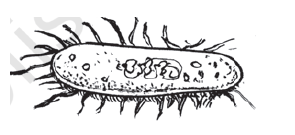# Q5. A photograph of a bacteria enlarged 50,000 times attains a length of 5 cm as shown in the diagram. What is the actual length of the bacteria? If the photograph is enlarged 20,000 times only, what would be its enlarged length?We can calculate it easily,

Given that for 50,000 times enlarged, the photograph of a bacteria attains a length of 5cm.

So, we have to calculate the actual length of the bacteria(assume it to be 'x'cm) that is when the photograph is enlarged to 1 time

Knowing that the microscope's zoom has a direct relation with the length of bacteria observed So, we get the relation ;

Solving the equation for x we get;

The actual length of the bacteria is  which is so small to be observed through naked eyes.

Now, calculating the length of bacteria when it the photograph is enlarged 20,000 times,

assume it as 'y'.

So, we get this relation

solving for y we get;

.

So, if the photograph is enlarged 20,000 times only then the enlarged length would be 2cm.

Exams
Articles
Questions